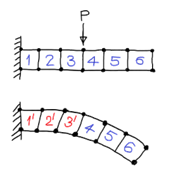Convergence Criteria

In order to achieve a monotonically convergent solution, the chosen displacement functions must satisfy the following criteria known as the Convergence Criteria.
1. Continuity requirement: The displacement functions should be continuous within an element.
2. The displacement field must represent the rigid body displacement of the element.
3. The displacement field must represent constant strain states of the element.
4. Compatibility requirement: The displacements must be compatible between adjacent elements.
5. The displacement functions must have the same number of generalized coordinates equal to the degrees of freedom of the element.
6. Geometric Invariance (Spatial Isotropy): The displacement functions should be independent of the local coordinate system.
The elements which satisfy the continuity and compatibility criteria are called Compatible or Conforming element. Otherwise, they are called as Non-ConformingThe elements which satisfy the 2nd and 3rd criteria are known as Complete.

Explanation:

Continuity criteria:
The displacement functions (field variables) should be continuous within an element. This requirement is easily satisfied by choosing continuous functions as interpolation functions. Since polynomials are inherently continuous, the polynomial type of interpolation functions satisfy this requirement.
The displacement field must represent the rigid body displacement of the element:
That is when the nodes are given such displacements corresponding to a rigid body motion, the element should not experience any strain and hence leads to zero nodal forces. The constant term in the polynomials used for the displacement models would usually ensure this condition.
Consider a cantilever beam under a concentrated load in the middle. As a result of this loading, deformation occurs only to the left of the load. The section of the beam to the right of the load experiences only rigid-body translations and rotations (constant displacements and Zero strain) i.e., no stresses and strains occur. Therefore, the element approximation functions must permit such behaviour. Complete polynomials satisfy these requirements.The displacement field must represent constant strain states of the element:
Suppose, we imagine the subdivision of a continuum into smaller and smaller elements. Then the nodes of the element may come quite close to one another. In such cases the variation of strains goes negligibly small giving rise to a constant strain. This requirement is satisfied by including a linear term in the polynomial approximation function.
Interelement compatibility requirement:
The displacements must be compatible between adjacent elements. The chosen displacement functions should have continuity within the element and also the displacements obtained through them should be compatible at the element interfaces. The adjacent elements must deform without any kinking, gaps or overlaps at the common edge between the two elements.
If the description of a function along an interelement boundary is specified uniquely by the degree of freedom along that boundary, then interelement continuity of that function is preserved in the joining of that element to a counterpart element along the same boundary.Geometric Isotropy (Geometric Invariance):
The displacement function or pattern should be independent of the local coordinate system.
One of the most important considerations in choosing the proper terms in the polynomial expansion is that element should have no preferred direction. That is the displacement shape will not change with a change in the local coordinate system. This property is known as Geometric Isotropy or Geometric Invariance.
Geometric Invariance is achieved if the polynomial includes all the terms. That is, the polynomial is a complete one. Invariance may be achieved if the polynomial is balanced in case all the terms cannot be included.